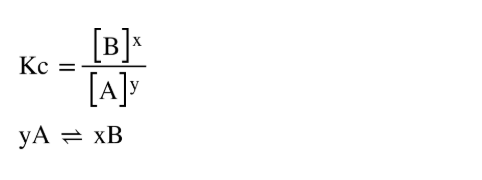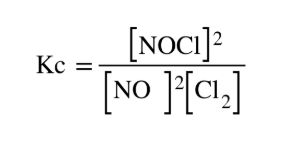# Problem: Consider the reaction between NO and Cl2 to form NOCl: 2NO(g) + Cl2(g) ⇌ 2NOCl(g)A reaction mixture at a certain temperature initially contains only [NO] = 0.61 M and [Cl2]= 0.61 M. After the reaction comes to equilibrium, the concentration of NOCl is 0.31 M. Find the value of the equilibrium constant (Kc) at this temperature.

###### FREE Expert Solution
• Kc expression can be written as:• Setting up the Kc expression for the reaction 2NO(g) + Cl2(g) ⇌ 2NOCl(g) :• To find Kc, we must calculate the concentration of each species at equilibrium by setting up an ICE table

92% (229 ratings)###### Problem Details

Consider the reaction between NO and Cl2 to form NOCl: 2NO(g) + Cl2(g) ⇌ 2NOCl(g)
A reaction mixture at a certain temperature initially contains only [NO] = 0.61 M and [Cl2]= 0.61 M. After the reaction comes to equilibrium, the concentration of NOCl is 0.31 M. Find the value of the equilibrium constant (Kc) at this temperature.